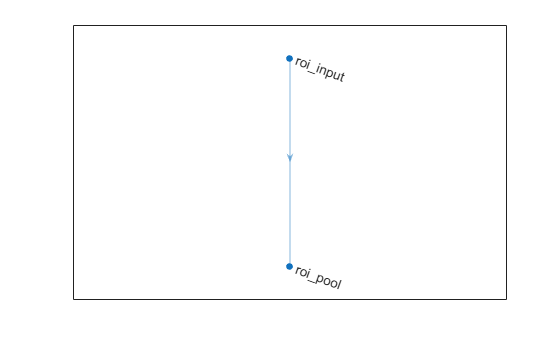# roiInputLayer

ROI input layer for Fast R-CNN

## Description

An ROI input layer inputs images to a Fast R-CNN object detection network.

## Creation

### Syntax

``layer = roiInputLayer``
``layer = roiInputLayer('Name',Name)``

### Description

``layer = roiInputLayer` creates an ROI input layer.`

example

````layer = roiInputLayer('Name',Name)` creates an ROI input layer and sets the optional `Name` property.```

## Properties

expand all

Layer name, specified as a character vector or a string scalar. To include a layer in a layer graph, you must specify a nonempty, unique layer name. If you train a series network with the layer and `Name` is set to `''`, then the software automatically assigns a name to the layer at training time.

Data Types: `char` | `string`

Number of outputs of the layer. This layer has a single output only.

Data Types: `double`

Output names of the layer. This layer has a single output only.

Data Types: `cell`

## Examples

collapse all

Create an ROI input layer.

`roiInput = roiInputLayer('Name','roi_input');`

Create an ROI max pooling layer with output size [4 4].

```outputSize = [4 4]; roiPool = roiMaxPooling2dLayer(outputSize,'Name','roi_pool');```

Add the layers to a LayerGraph.

```lgraph = layerGraph; lgraph = addLayers(lgraph,roiInput); lgraph = addLayers(lgraph,roiPool);```

Specify that the output of the ROI input layer is the `'roi'` input of the ROI max pooling layer.

```lgraph = connectLayers(lgraph,'roi_input','roi_pool/roi'); plot(lgraph)```Introduced in R2018b